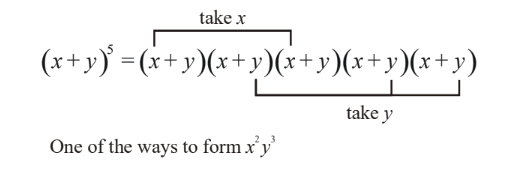# Binomial Theorem For Positive Integer Indices

Go back to  'Binomial Theorem'

Let us now consider more formally the binomial theorem. We need to expand $${\left( {x + y} \right)^n}$$ , where x, y are two arbitrary quantities, but n is a positive integer.

In particular, if we write

${\left( {x + y} \right)^n} = \left( {x + y} \right)\left( {x + y} \right)\left( {x + y} \right)...\left( {x + y} \right) \qquad \left( {n\,\,{\rm{times}}} \right)\,\,\,\,\,\,\,\,\,\,\,\,\,\,\,\,\,\,\,\,\,\,\,\,\,\,\,\,\,\,\,\,\,\,\,\,\,\,\,\,\,\,\,\,\,\,\,\,\,\,\,\,\,\,\,\,...{\rm{ }}\left( 1 \right)$

we need to find out the coefficient of $${x^i}{y^j}$$ . Note that $$i + j$$ must always equal n, so that we can write a general term of the expansion (without the coefficient) as $${x^r}{y^{n - r}}$$ so that $$0 \le r \le n$$ .

Now, to find the coefficient of $${x^r}{y^{n - r}}$$ , note that we need the quantity x, r times, while y is needed n r times. Thus, in (1), $${x^r}{y^{n - r}}$$ will be formed whenever x is ‘contributed’ by r of the binomial terms, while y is ‘contributed’ by the remaining n - r of the binomial terms. For example, in the expansion of $${\left( {x + y} \right)^5}$$ , to form $${x^2}{y^3}$$ , we need x from 2 terms and y from 3:Fig. 23

How many ways are there to form $${x^2}{y^3}$$ ? In other words, how many times will $${x^2}{y^3}$$ be formed? The number of times $${x^2}{y^3}$$ is formed is what is the coefficient of $${x^2}{y^3}$$ . That number, which would be immediately obvious to the alert reader, is simply $$^5{C_2}$$ . Why?  Because this is the number of ways in which we can select any 2 binomial terms from 5. These 2 terms will contribute x. The remaining will automatically contribute y.

In the general case of $${\left( {x + y} \right)^n}$$ , we see that the coefficient of $${x^r}{y^{n - r}}$$ would be $$^n{C_r}$$ . (which is infact the same as $$^n{C_{n - r}}$$ ). Thus, the general binomial expansion is

$\Rightarrow \qquad \fbox{{{{\left( {x + y} \right)}^n} = \sum\limits_{r = 0}^n {{\,^n}{C_r}} \,{x^{n - r}}{y^r}}}$

The coefficients $$^n{C_i}$$ are called the binomial coefficients, for a reason that should now be obvious.

Note that the $${\left( {i + 1} \right)^{{\rm{th}}}}$$ coefficient in this expansion is $$^n{C_i}$$ , which now explains the relation

${T_{n,\,i + 1}} = {T_{n - 1,i}} + {T_{n - 1,\,i + 1}}$

we observed in the Pascal triangle; this relation simply corresponds to

$^n{C_i} = {\,^{n - 1}}{C_{i - 1}} + {\,^{n - 1}}{C_i}$

Also, the binomial coefficients of terms equidistant from the beginning and the end are equal, because we have $$^n{C_r} = {\,^n}{C_{n - r}}$$ . The general term of expansion, $$^n{C_r}{x^{n - r}}{y^r}$$ , is the (r + 1) th  term from the beginning of the expansion and is conventionally denoted by $${T_{r + 1}}$$ , i.e.

${T_{r + 1}} = {\,^n}{C_r}\,{x^{n - r}}{y^r}$

Since we have (n + 1) terms in the general expansion, we see that if n is even, there will be an odd number of terms, and thus there will be only one middle term, which would be $$^n{C_{n/2}}\,{x^{n/2}}{y^{n/2}}$$ . For example,

${\left( {x + y} \right)^4} = {x^4} + 4{x^3}y + \mathop {6{x^2}{y^2}}\limits_{\scriptstyle\,\,\,\,\,{\rm{only}}\,{\rm{one}} \atop\scriptstyle{\rm{middle}}\,{\rm{term}}} + 4x{y^3} + {y^4}$

On the other hand, if n is odd, then there will be an even number of terms in the expansion, and thus there will be two middle terms, namely \begin{align}^n{C_{\frac{{n - 1}}{2}}}\,\,{x^{\frac{{n + 1}}{2}}}\,{y^{\frac{{n - 1}}{2}}}\,\,{\rm{and}}\,{\,^n}{C_{\frac{{n - 1}}{2}}}\,\,{x^{\frac{{n - 1}}{2}}}\,{y^{\frac{{n + 1}}{2}}}\end{align}

For example;

${\left( {x + y} \right)^5} = {x^5} + 5{x^4}y + \mathop {10{x^3}{y^2} + 10{x^2}{y^3}}\limits_{{\rm{Two}}\,\,{\rm{middle}}\,\,\,{\rm{terms}}} + 5x{y^4} + {y^5}$

Example – 1

Find the middle term(s) in the expansion of $${\left( {x - \frac{1}{x}} \right)^9}$$

Solution:    Since n = 9, there will be 10 terms in the expansion, which means that there will be 2 middle terms in the expansion, the 5 th  and the 6 th :

$\begin{array}{l}{T_5} = {\,^9}{C_4}{\left( x \right)^{9 - 4}}\left( {\frac{{ - 1}}{x}} \right)_{}^4 = 126\,x\\{T_6} = {\,^9}{C_5}{\left( x \right)^{9 - 5}}{\left( {\frac{{ - 1}}{x}} \right)_5} = \frac{{ - 126}}{x}\end{array}$

Example – 2

Is there any term in the expansion of $${\left( {\frac{{2{x^2}}}{5} - \frac{1}{{\sqrt x }}} \right)^{10}}$$ that will be independent of x?

Solution:    The general term in the expansion is

$\begin{array}{l}{T_{r + 1}} = {\,^{10}}{C_r}{\left( {\frac{{2{x^2}}}{5}} \right)^{10 - r}}{\left( {\frac{{ - 1}}{{\sqrt x }}} \right)^r}\\\,\,\,\,\,\,\,\,\, = {\,^{10}}{C_r}{\left( {\frac{2}{5}} \right)^{10 - r}}{\left( { - 1} \right)^r}\,\,{x^{20 - 2r - \frac{r}{2}}}\\\,\,\,\,\,\,\,\,\, = {\,^{10}}{C_r}{\left( {\frac{2}{5}} \right)^{10 - r}}{\left( { - 1} \right)^r}\,\,{x^{20\, - \frac{{5r}}{2}}}\end{array}$

Thus, for the term that is independent of x, we have

$\begin{array}{l}\,\,\,\,\,\,\,\,\,\,\,\,\,\,\,\,20 - \frac{{5r}}{2} = 0\\ \Rightarrow \qquad r = 8\end{array}$

Thus, the term free of x is the 9 th  term given by

${T_9} = {\,^{10}}{C_8}{\left( {\frac{2}{5}} \right)^2}{\left( { - 1} \right)^8} = \frac{{36}}{5}$